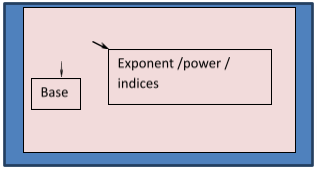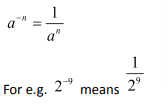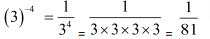In this article, we will see what exponents really are and go through some tips to solve the exponential expressions. Exponents also go by the name of powers and indices. So in case you find any of these words used interchangeably in various texts, be rest assured they refer to the same.

Basic Definition of Exponents

Exponents are a method that is used to express products of the same number.

How would you write: 2 x 2 x 2 x 2 x 2 x 2 x 2 x 2 x 2?

Hard to repeat the above number in calculations, isn’t it?

Well, exponents provide us the solution to the above problem. The above number simply becomes: 29For the expression AN:

• A is called the base
• N is called the exponent

It is generally read as A to the power N.

Essentially, we can sayThe reverse of the exponent form is the product form.

Example: How would you writein product form?

Solution: We know=5x5x5

Negative exponent:  As we have seen above, exponent refers to essentially multiplication of a number with itself. In the same way, negative exponent means division. Negative exponent is the inverse of exponent.So, whenever you find negative exponent, flip it down and then you can use it exactly like exponents or positive exponents.

Let us take up an example of what a negative exponent is.

Example:  What is the value of (3)–4

Solution:Example:  What is the value of (–2)–5  ?

Solution:Useful results for Exponents

Now let us take up some useful results for exponents that we can use to solve questions. These rules are essentially based on basic concepts and if you understand these rules, you go a long way in solving questions based on exponents.

Tooltip 1: Exponents in the case of negative numbers

You can represent exponents for negative numbers as:For example,

(i)(ii)Tooltip 2: Exponents for fractions

Letbe any rational number and n be a positive integer. Then,times =.

Thus,for every positive integer n.

Example:  Elaborate :-Solution:   We can write both numerator and denominator in product form separately.=Example: Writein exponent form.

Solution: Count numbers in numerator and denominator and write them in their respective powers.

SoTooltip 3: Another rule for exponents related to fractions

Letbe any rational number and n be a positive integer.

Then, we define,.

EXERCISE:

Question 1: Evaluatea. 4/25

b. 8/125

c. 8/25

d. 16/125

Solution:   We know

Hence, option (b)Question 2:  Evaluatea. -27/125

b. 27/125

c. 27/625

d. -9/125

Solution:  We knowHence, option (a)

Question 3: Evaluate

a. 125/27

b. 27/125

c. -125/27

d. -27/125

Solution:   We knowHence, option (b)

Question 4: Evaluate 5-2

a. 25

b. -25

c. 1/25

d. -1/25

Solution:  It is an example of negative exponent

AsSoHence, option (c)

Question 5: Evaluate

a. 1/25

b.  -25

c. -1/25

d. 25